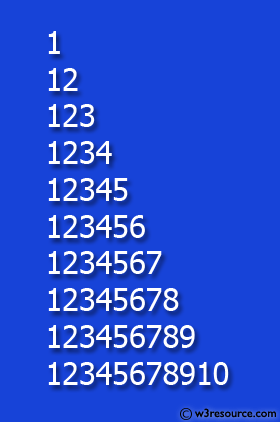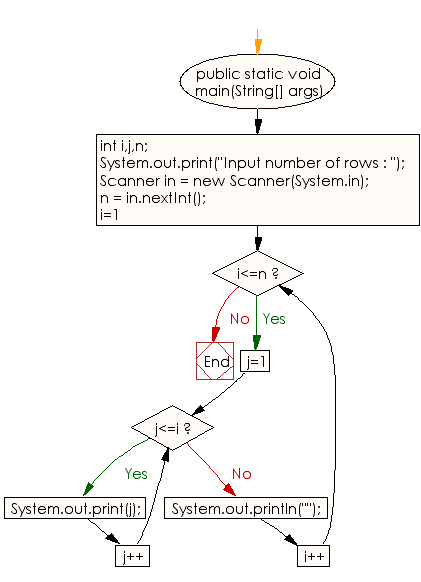﻿ Java exercises: Display the pattern like right angle triangle with a number - w3resource

# Java Conditional Statement Exercises: Display the pattern like right angle triangle with a number

## Java Conditional Statement: Exercise-16 with Solution

Write a program in Java to display the pattern like right angle triangle with a number.

Test Data
Input number of rows : 10

Pictorial Presentation:Sample Solution:

Java Code:

``````import java.util.Scanner;
public class Exercise16 {

public static void main(String[] args)

{
int i,j,n;
System.out.print("Input number of rows : ");
Scanner in = new Scanner(System.in);
n = in.nextInt();

for(i=1;i<=n;i++)
{
for(j=1;j<=i;j++)
System.out.print(j);

System.out.println("");
}
}
}
```
```

Sample Output:

```Input number of rows : 10
1
12
123
1234
12345
123456
1234567
12345678
123456789
12345678910
```

Flowchart:Java Code Editor: